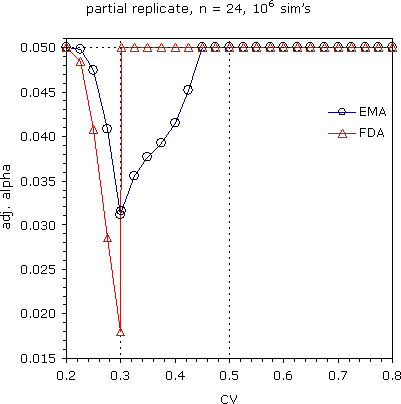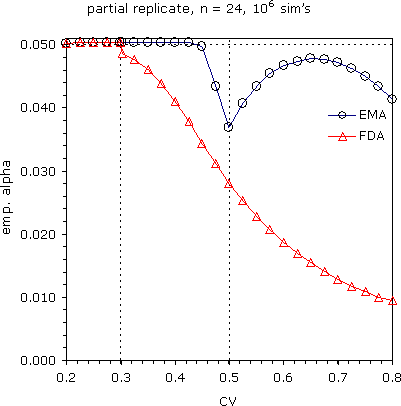Dear all!

» this behavior may be due to an adaptive choice of a statistical method in face of the data without any "adjustment" for this adaptation.

Yep. The acceptance range is data-driven (random variable dependent on CVWR).

» PS.: no idea at the moment how to account for this.

library(PowerTOST)
reg   <- "EMA"   # "EMA" for scABEL or "FDA" for RSABE
CV    <- 0.300   # intra-subject CV of reference
n     <- 24      # total sample size
# in case of imbalanced sequences use a vector:
# e.g., c(n1,n2,n3)
des   <- "2x3x3" # partial: "2x3x3" full: "2x2x4"
print <- FALSE   # intermediate results
if (reg == "EMA") { # scABEL
ifelse (CV <= 0.5, GMR <- exp(0.7601283*CV2se(CV)),
GMR <- exp(0.7601283*CV2se(0.5)))
if (CV <= 0.3) GMR <- 1.25
} else {        # RSABE
ifelse (CV > 0.3, GMR <- exp(0.8925742*CV2se(CV)), GMR <- 1.25)
}
nsims <- 1e6
prec  <- 1e-8     # precision of bisection algo
x     <- 0.05     # target alpha
sig   <- binom.test(x*nsims, nsims,
alternative='less')$conf.int # significance limit of sim’s nom <- c(0.001, x) # from conservative to target alpha lower <- min(nom); upper <- max(nom) delta <- upper - lower # interval ptm <- proc.time() # start timer iter <- 0 while (abs(delta) > prec) { # until precision reached iter <- iter + 1 x.new <- (lower+upper)/2 # bisection if (reg == "EMA") { pBE <- power.scABEL(alpha=x.new, theta0=GMR, CV=CV, n=n, design=des, nsims=nsims) } else { pBE <- power.RSABE(alpha=x.new, theta0=GMR, CV=CV, n=n, design=des, nsims=nsims) } if (print == TRUE) cat(iter, x.new, pBE, "\n") if (abs(pBE - sig) <= prec) break # precision reached if (pBE > sig) upper <- x.new # move limit downwards if (pBE < sig) lower <- x.new # move limit upwards delta <- upper - lower # new interval } if (print) cat("run-time:", round((proc.time()-ptm),1), "seconds iterations:", iter, "\n") cat("regulator:", reg, " CV:", CV, " n:", n, " design:", des, "\ntarget alpha:", x, " adjusted alpha:", x.new, " pBE:", pBE, "\n") CV adj alpha pBE_scABEL adj alpha pBE_RSABE 0.200 0.050000 0.050220 0.050000 0.050153 0.225 0.049730 0.050360 0.048467 0.050359 0.250 0.047370 0.050359 0.040790 0.050360 0.275 0.040833 0.050360 0.028606 0.050359 0.300 0.031124 0.050359 0.017999 0.050360 0.302 0.031612 0.050359 0.050000 0.048548 0.325 0.035545 0.050360 0.050000 0.047741 0.350 0.037636 0.050360 0.050000 0.046156 0.375 0.039190 0.050360 0.050000 0.043941 0.400 0.041417 0.050360 0.050000 0.041041 0.425 0.045221 0.050360 0.050000 0.037799 0.450 0.050000 0.049795 0.050000 0.034401 0.475 0.050000 0.043420 0.050000 0.031150 0.500 0.050000 0.036960 0.050000 0.028097 0.525 0.050000 0.040644 0.050000 0.025383 0.550 0.050000 0.043412 0.050000 0.022875 0.575 0.050000 0.045423 0.050000 0.020699 0.600 0.050000 0.046647 0.050000 0.018660 0.625 0.050000 0.047379 0.050000 0.016948 0.650 0.050000 0.047754 0.050000 0.015457 0.675 0.050000 0.047698 0.050000 0.014135 0.700 0.050000 0.047192 0.050000 0.012861 0.725 0.050000 0.046282 0.050000 0.011799 0.750 0.050000 0.045020 0.050000 0.010865 0.775 0.050000 0.043338 0.050000 0.010057 0.800 0.050000 0.041320 0.050000 0.009425Take my code with a grain of salt. Cases where no adjustment is necessary agree with Detlew’s results. Note: Any result <0.05035995 is not significantly >0.05 in 106 simulations. However, if you want to get a more conservative estimate replace sig <- binom.test(x*nsims, nsims, alternative='less')$conf.int

by

sig   <- 0.05

Edit: Much faster code in this post.

Dif-tor heh smusma 🖖
Helmut SchützThe quality of responses received is directly proportional to the quality of the question asked. 🚮
Science QuotesIng. Helmut Schütz